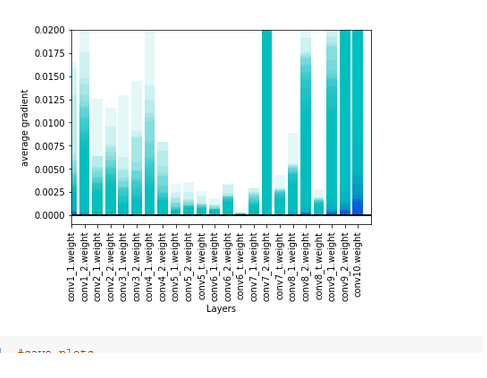Hello. I have a Unet network for segmentation. Using dice loss the net is learning. But when I use Crossentropy, after one epoch the loss is constant. I ve searched on internet and i ve found a function to check gradients, and after 3 epochs they become 0. Can someone help me with some ideas?
I already use Relu, and init the conv2d with xavier.

You can try to use leaky relu. I used it with unet and never had problems.

Ok, but do you have an explanation, why with dice loss is no problem and with crossentropy it is?

Hmm it’s difficult to say. In theory cross entropy should has better gradient properties. You can find a post comparing both here https://becominghuman.ai/investigating-focal-and-dice-loss-for-the-kaggle-2018-data-science-bowl-65fb9af4f36c.

Anyway could you paste couple of loss plots?. If you report small gradients at an early stage I assume there exist a smooth plateau in you loss for a high loss value. If you had big gradients but no convergence you would have oscillating loss and probably it’s not the case. Have you tried different set of initializations/lr schemes?

I have constant loss.
For example for adam optimiser with:
lr = 0.01 the loss is 25 in first batch and then constanst 0,06x and gradients after 3 epochs. But 0 accuracy.

lr = 0.0001 the loss is 25 in first batch and then constant 0,1x and gradients after 3 epochslr = 0.00001 the loss is 1 in first batch and then after 6 epochs constantand gradients i dont know if thery are too smallCan you please share me what function you used to check gradients?
It is a little hard on my environment to work with tensorboard.

``````def plot_grad_flow(named_parameters):
'''Plots the gradients flowing through different layers in the net during training.
Can be used for checking for possible gradient vanishing / exploding problems.

Usage: Plug this function in Trainer class after loss.backwards() as
layers = []
for n, p in named_parameters:
if(p.requires_grad) and ("bias" not in n):
layers.append(n)
plt.hlines(0, 0, len(ave_grads)+1, lw=2, color="k" )
plt.ylim(bottom = -0.001, top=0.02) # zoom in on the lower gradient regions
plt.xlabel("Layers")
I found this on forum
``````
2 Likes

I does not look like you are getting gradient vanishing. In fact it is difficult with unet due to skip connections. It also looks like that even decreasing lr you are arriving to same local minima, thus, a bigger LR at early stage does not help not to fall in there. Could be a matter of trying different initialization/optimizers in order to see if the lead to another place?

Anyway, do you have a healthy dataset with balanced amount of classes?

It’s a segmantation task. I have 2.5k images for traning. A gts containg 90% background and 10% tumor, or less I think

So you are using BCE with logits rather than crossentropy right? Have you tried to penalize the weight of background? It sounds like dice loss works as its kind of IoU but with crossentroypy model starts predicting all as background and it would be accurate loss-wise.

I only use dice loss. Is there a loss that penalize weigth, bootstrapping loss no?I havent use it yet.

You started the post saying

As far as I understand, your problem was crossentropy does not train but dice does. So one possible reason is that you should use BCE rather than crossentropy (as it is a binary classification, background vs foreground) and to penalize the weight with wich background contributes to the loss as it is 90% cases (thus, net will be biased to predict background).

Hello. I dont know if is a problem with the arhitecture.
But I have a new problem with a loss function, I found an implementation for bootstrap loss`class SoftBootstrappingLoss(nn.Module):
“”"
Loss(t, p) = - (beta * t + (1 - beta) * p) * log§
“”"
def init(self, beta=0.9, reduce=True):
super(SoftBootstrappingLoss, self).init()
self.beta = beta
self.reduce = reduce

``````def forward(self, input, target):
target = target.long()
target = target.view(-1, size_image, size_image)
# cross_entropy = - t * log(p)
beta_xentropy = self.beta * F.cross_entropy(input, target, reduce=False)

# second term = - (1 - beta) * p * log(p)
bootstrap = - (1.0 - self.beta) * torch.sum(F.softmax(input, dim=1) * F.log_softmax(input, dim=1), dim=1)

if self.reduce: